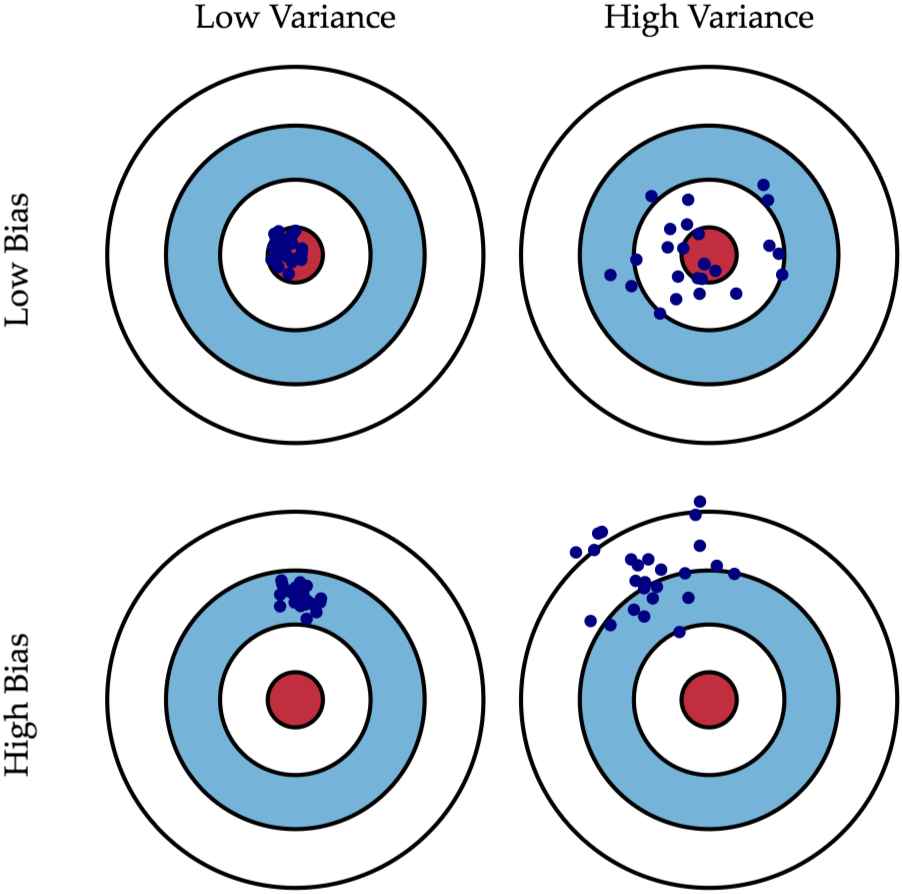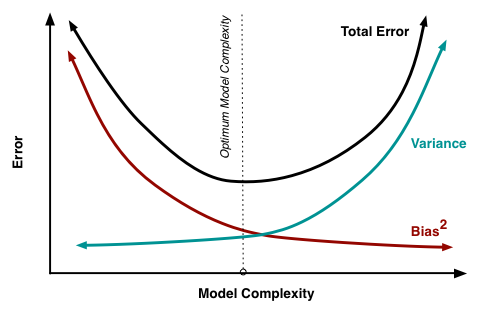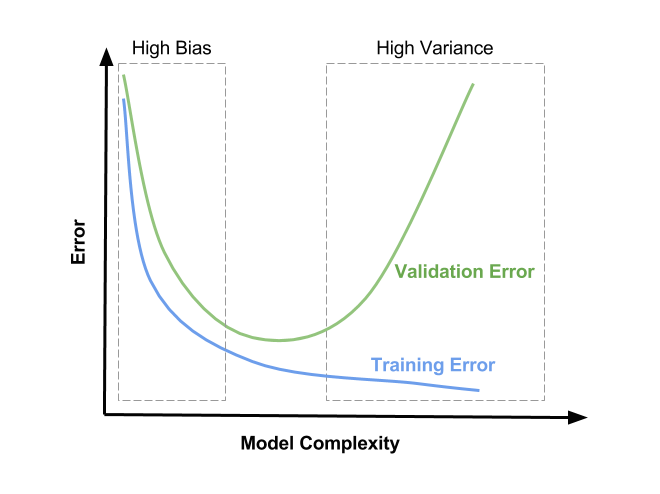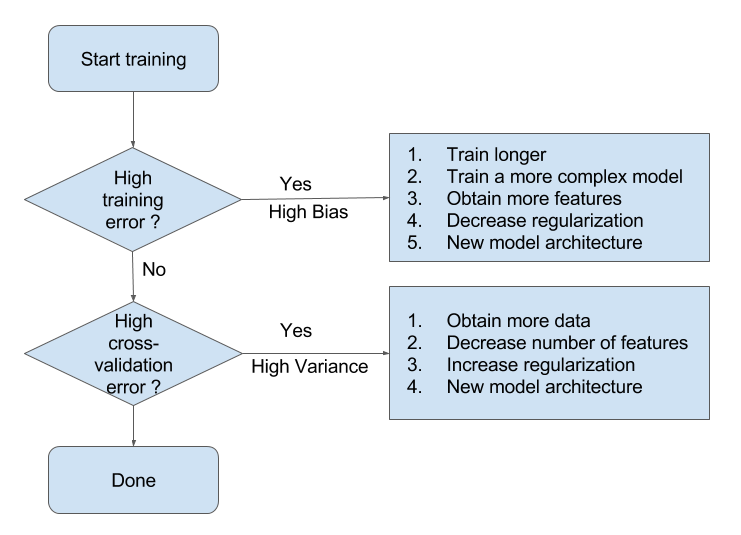——Liam Huang

## 定义

### 数学上定义

$$y = f(X) + \epsilon.$$

• 偏差（bias）描述的是通过学习拟合出来的结果之期望，与真实规律之间的差距，记作 $\text{Bias}(X) = E[\hat f(X)] - f(X)$。
• 方差（variance）即是统计学中的定义，描述的是通过学习拟合出来的结果自身的不稳定性，记作 $\text{Var}(X) = E\Bigl[\bigl(\hat f(X) - E[\hat f(X)]\bigr)^{2}\Bigr]$。

\begin{equation} \begin{aligned} \text{Err}(X) &{}= E\Bigl[\bigl(y - \hat f(X)\bigr)^2\Bigr] \\ &{}= E\Bigl[\bigl(f(X) + \epsilon - \hat f(X)\bigr)^2\Bigr] \\ &{}= \left(E[\hat{f}(X)]-f(X)\right)^2 + E\left[\left(\hat{f}(X)-E[\hat{f}(X)]\right)^2\right] +\sigma_\epsilon^2 \\ &{}= \text{Bias}^2 + \text{Variance} + \text{Random Error}. \end{aligned} \label{eq:err-comp} \end{equation}

### 直观的图示http://scott.fortmann-roe.com/docs/BiasVariance.html

## 举个栗子## 权衡之术

### 最佳平衡点的数学表述

• 选定一个算法；
• 调整算法的超参数；
• 以某种指标选择最合适的超参数组合。http://scott.fortmann-roe.com/docs/BiasVariance.html

\begin{equation} \newcommand{\dif}{\mathop{}\!\mathrm{d}} \frac{\dif\text{Bias}}{\dif\text{Complexity}} = - \frac{\dif\text{Variance}}{\dif\text{Complexity}} \label{eq:sweet} \end{equation}

### 过拟合与欠拟合的外在表现http://www.learnopencv.com/bias-variance-tradeoff-in-machine-learning/

• 当模型处于欠拟合状态时，训练集和验证集上的误差都很高；
• 当模型处于过拟合状态时，训练集上的误差低，而验证集上的误差会非常高。

## 处理欠拟合与过拟合• 增加模型的迭代次数；
• 更换描述能力更强的模型；
• 生成更多特征供训练使用；
• 降低正则化水平。

### 过拟合

• 扩增训练集；
• 减少训练使用的特征的数量；
• 提高正则化水平。